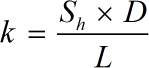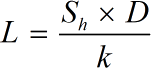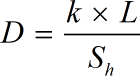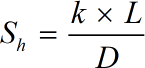Request a Tool

# Sherwood Number Calculator

Sherwood Number is termed as a dimensionless number which is used in the study of mass- transfer operation.

#### Output

Mass Transfer Coefficient
0

#### Formula• k = Mass Transfer Coefficient
• L = Characteristic Length
• D = Diffusion Coefficient
• Sh = Sherwood Number

#### Defination / Uses

The Sherwood Number represents the ratio of convective to diffusive mass transport. The Sherwood Numbers is defined as a relationship between a mass transfer coefficient and a characteristic length, such as pipe diameter and a coefficient of diffusion. Sherwood Number is named in the honor of Thomas Kilgore Sherwood.

#### Output

Characteristic Length
0

#### Formula• L = Characteristic Length
• k = Mass Transfer Coefficient
• D = Diffusion Coefficient
• Sh = Sherwood Number

#### Defination / Uses

Characteristic length is a crucial dimension in physics that specifies the scale of a physical system. Characteristic length is defined in computational mechanics to force localization of a stress softening constitutive equation. An integration point is referred to the length.

#### Output

Diffusion Coefficient
0

#### Formula• D = Diffusion Coefficient
• L = Characteristic Length
• k = Mass Transfer Coefficient
• Sh = Sherwood Number

#### Defination / Uses

The Sherwood Number represents the ratio of convective to diffusive mass transport. A relationship between a mass transfer coefficient and a characteristic length, such as pipe diameter and coefficient of diffusion, is defined as the Sherwood Numbers. Thomas Kilgore Sherwood was honoured with the Sherwood Number.

Sherwood Number
0

#### Formula• Sh = Sherwood Number
• D = Diffusion Coefficient
• L = Characteristic Length
• k = Mass Transfer Coefficient

#### Defination / Uses

The Sherwood Number represents the ratio of convective to diffusive mass transport. The Sherwood Numbers is defined as a relationship between a mass transfer coefficient and a characteristic length, such as pipe diameter and a coefficient of diffusion. Sherwood Number is named in the honor of Thomas Kilgore Sherwood.

### How to use this calcultor?

There are only two basic steps.

• First select the option from dropdown. What you need to calculate.
• Then add the other values in their corresponding fields.